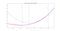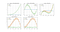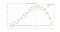# Gauss-Newton algorithm implementation from scratch

Python implementation of the powerful Gauss-Newton optimization method. All the code can be found from my GitHub repo.

# Background

Here I’m going to present an implementation of the gradient-based optimization algorithm, Gauss-Newton. The implementation is done from scratch using only NumPy as a dependency.

I will not go deep into theory or mathematics. For that purpose, there is plenty of good sources available, e.g. Wikipedia. Instead, I will try to provide a more intuitive explanation that is based on a geometric interpretation of the method.

# Small dive into the theory

You might already know the well-known gradient-based optimization method, gradient descent. Gradient descent calculates derivative (or gradient in multidimensional case) and takes a step in that direction. Gauss-Newton method goes a bit further: it uses curvature information, in addition to slope, to calculate the next step. The method takes a big step if the curvature is low and small step if the curvature is high. For this reason, in some cases, it can converge to the minimum in much fewer iterations than gradient descent.

Gauss-Newton is actually an approximation of a more general method called Newton’s method which is based on approximating function with second-order Taylor expansion. I think that Newton’s method is best illustrated geometrically in 1-dimensional case: Newton’s method will approximate the actual function locally with quadratic function (using local first and second derivative) around current guess and then proceeds to the extreme of that quadratic function. This is repeated until the converge criterium is filled. In a multidimensional case, the derivative is replaced with the gradient (matrix of first partial derivatives) and the second derivative with the Hessian matrix (matrix of second partial derivatives).Newton’s method visualized in 1-dimensional case. The method approximates the function (red) locally by fitting quadratic function (blue) around the current estimate (blue dashed vertical line) and proceeds to the extreme of the quadratic fit. Here, the method has proceeded from x0 -> x1 -> x2 and is already close to the actual minimum.

In practice, Newton’s method is rarely used as it is because Hessian matrix can be challenging or expensive to compute. For more detailed information, you can check for example this page. At this point, Gauss-Newton comes to rescue. Gauss-Newton makes one more approximation: instead of calculating Hessian matrix directly, it approximates this matrix with so called Gauss–Newton Hessian matrix and thus avoids problems that are related to calculation of exact Hessian matrix.

For implementation purposes, we actually need only one simple equation, Gauss-Newton update rule. Gauss-Newton optimization proceeds iteratively by updating coefficient values (values we want to solve) using the following Gauss-Newton update rule:

βᵏ⁺¹ = βᵏ + J⁺ r(βᵏ)

where

βᵏ⁺¹ = updated coefficient values

βᵏ = current estimate for coefficients values

J⁺ = pseudoinverse of the Jacobian matrix, where Jᵢⱼ = ∂rᵢ(βᵏ) / ∂βⱼ

r(βᵏ) = residual vector, calculated with the current estimate for coefficients

# Implementation

Enough theory and let’s do some coding. Here is the problem that needs to be solved:

Given response vector y, dependent variable x and fit function f,
Minimize sum(residual²) where residual = f(x, coefficients) -y.

The full implementation of GNSolver can be found below. We use OOP and define a class called GNSolver. GNSolver takes a user-defined fit function as input. Here, the fit function is defined so that y =fit_function(x, coefficients), where y is response vector, x is the dependent variable, and coefficients is a vector that needs to be solved. The constructor takes these parameters as arguments and stores them as member variables.

The fit method takes independent variable x and response y as input. Based on the initial guess for coefficients, the function calculates the initial residual vector which is the difference between estimated response and actual response. Then the Jacobian matrix is calculated and it used to update our coefficients estimates using the Gauss-Newton update rule. This process is repeated iteratively until convergence criterium is filled or the maximum number of iterations has been reached. As a solution, the method returns fitted coefficients.

We also define few getter methods (get_residual, get_estimate) that can be used to retrieve residual and estimated response after fitting.

Note that in the implementation the Jacobian matrix is calculated numerically. If you care about performance, you should calculate this analytically (and also, use some other language than Python). The nice thing about numerical calculation though is that it works with any fit function without modifications.

# Gauss-Newton in action: curve fitting example

For testing purposes, let’s define a function that is a combination of a polynomial and periodic sine function.

y = c₀ × x³ + c₁ × x² + c₂ × x + c₃ + c₄ × sin(x)

Let’s use this same function to generate data and then fit the coefficients using GNSolver. To make the job more realistic, let’s also add some random amplitude noise. Let’s also make a really bad initial guess: random values that are about 10⁶ times larger than there actual values.

The visualization below shows the result. The iteration converges quickly; after 5 iterations RMSE difference between iterations is smaller than 0.0001 % and iteration is terminated. The fitted curve matches well with the actual response and the remaining residual is due to added amplitude noise.Gauss-Newton fit iterations. The fit converges after 5 iterations.Gauss-Newton fit to noisy signal (orange) that is a combination of a polynomial and periodic sine function. The fitted signal (green) aligns well with the original noiseless signal (blue).

Here is the code of the sample: